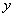This page has been robot translated, sorry for typos if any. Original content here.

# Model of economics - Vіtlіnsky V.V.

## 5.5.2. Bagatofactory virobnich functions

In the economic and mathematical model, there is a wide variety of bagatofactory virobnich functions.

One of the most rational ways to switch between two-factor and one-way functions in such a field.

Rosglyano two-factor function:

y = j1 ( x 1, x 2). (5.8)

Argument x 2 values ​​of functions we can see as far as explications are indicated, which is also necessary to lay down the same two internal officials x 3, x 4:

x 2 = j2 ( x 3, x 4),

de j2 - deyaka functions. We represent the formula (5.8), which is trifactor function

y = j1 ( x 1, j2 ( x 3, x 4)),

що turn the indicatorenter arguments x 1, x 3, x 4. This process can be promoted, impaired, secrecy, but x 3, have your own account, to lay down the original dekyak officials.

At the outside view: ( n - 1) two-factor functions j1 ( x 1, x 2), j2 ( x 3, x 4), j n –1 ( x 2 n –3, x 2 n –2), then distance p- factor function:

y = f ( x 1, ..., xn )

at the result of the last substitution ikh. The operation of such a substitution (superposition) is the most obvious economical sense: another argument, on the application of two-factor functions, one after another to be presented at the sight of the other types of indicators of lower (detailed) rvn. It’s not important to translate such power of operation superposition:

a) i j1, ..., j n –1 - non-falling functions, then f - also non-falling functions;

b) for example, j2, ..., j n –1 is a linear-one-function func- tion, and j1 is a one-function func- tion of a single-function step g, then f is a single-function func- tion of a single-function step g;

c) it is j1, ..., j n - that the function has fallen away, then f - the function has fallen away.

From now on, it’s two-factorial functions j1, ..., j n –1 є neoclassical, then it is eliminated as a result of their superposition function f as well as neoclassical.

For viral functions, there are n fair ones, firm, as a rule, show what a class of functions is, giving the supervisor a superposition of any two-factor functions, є to achieve wide. Strictly conveyed, in order to ensure that the function is uninterrupted, f ( x 1, ..., xn ) is displayed n the last (for mind n ? 4) but can be served at the supervisor's view of the continuous functions of the three. At my house, the skin is uninterrupted in function of the three winter months, but the superposition of the two functions is completely different. Vidomo is also the same, be-yak uninterrupted function of the two functions is possible with the given precision of approximation of a superposition of uninterrupted functions of the same function y = x 1 + x 2.

Transition and okremі characteristics of deyaky classes of bagatofactor viral functions are induced at the lowest level * 2.

* 2: {Kleiner G. B. Production functions: Theory, methods, application. - M .: Finance and statistics, 1986.}